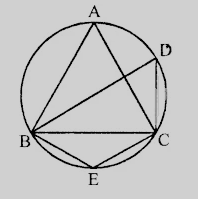"
">

# In the figure, if $ABC$ is an equilateral triangle. Find $\angle BDC$ and $\angle BEC$."

Given:

$ABC$ is an equilateral triangle.

To do:

We have to find $\angle BDC$ and $\angle BEC$.

Solution:

$\triangle ABC$ is an equilateral triangle.

This implies,

$\angle A = 60^o$

$\angle BAC$ and $\angle BDC$ are in the same segment.

Therefore,

$\angle BAC = \angle BDC = 60^o$

$BECD$ is a cyclic quadrilateral.

This implies,

$\angle BDC + \angle BEC = 180^o$

$60^o + \angle BEC = 180^o$

$\angle BEC = 180^o-60^o= 120^o$

Hence $\angle BDC = 60^o$ and $\angle BEC = 120^o$.

Updated on: 10-Oct-2022

49 Views